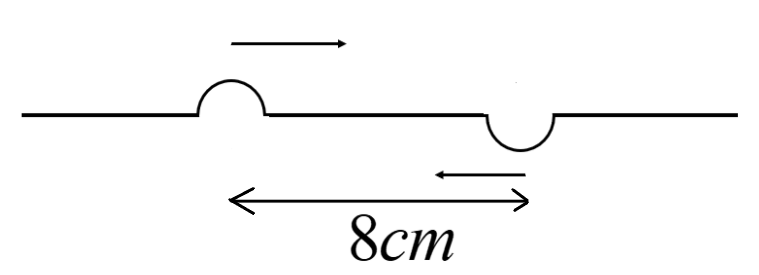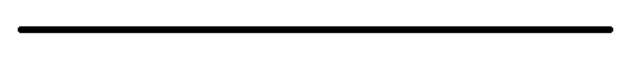QuestionAnswers

# Two pulses in a stretched string whose centres are initially $8cm$ apart are moving towards each other as shown in the figure. The speed of each pulse is $2cm/s$. After $2$ seconds the total energy of pulses will bea. Zerob. Purely kineticc. Purely potentiald. Partly kinetic and partly potentialVerified
91.8k+ views
Hint: To solve the given question, we need to calculate the speed for each pulse separately and by using the principle of superposition we are able to answer.

Formula used:
The distance can be calculated by using:
$\Rightarrow d = vt$
Where, $d$ is the distance, $v$ is the speed and $t$ is the time.

It is given that the two pulses in a stretched string in which the centres are initially $8cm$ apart are moving towards each other. The speed of each pulse is $2cm/s$.
We can solve the given problem with the help of the distance formula. We need to calculate the speed formula for each impulse separately.
Consider the upper impulse as $I$. We can calculate the distance for $I$. That is,
$\Rightarrow I \to d = vt$
The value of speed is two and time is two. We can substitute,
$\Rightarrow d = 2 \times 2$
$\Rightarrow d = 4cm$
Consider the upper impulse as $II$. We can calculate the distance for $II$. That is,
$\Rightarrow II \to d = vt$
The value of speed is two and time is two. We can substitute,
$\Rightarrow d = 2 \times 2$
$\Rightarrow d = 4cm$
Hence after $2s$ there will be overlap with each other.

We can apply the superposition principle. The principle of the super position, states that every charge in space creates an electric field at every point and is also independent of other charges in that given medium.
According to this principle the spring will be straight and it will not have any distortion. The position of the string is:Now we can calculate the total energy for the pulses. The total energy can be calculated as the sum of the kinetic and potential energy. That is,
$\Rightarrow T.E = K.E + P.E$
The value of potential energy is zero, in the question they mentioned about the moving of the string. Therefore, only kinetic energy will be acting. The total energy will have,
$\Rightarrow T.E = K.E + 0$
$\therefore T.E = K.E$
From this we can understand that the total energy of pulses will be purely kinetic energy.

Hence, the correct answer is option (B).

Note: The potential energy is measured as zero because the amplitude value in potential energy is zero, because after 2 seconds the string will become straight as the pulses will overlap and as there is no potential energy the entire energy will be kinetic energy.